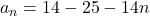Use the initial term and the recursive formula to find an explicit formula for the sequence an. Write your answer in simplest form.

Question

Use the initial term and the recursive formula to find an explicit formula for the sequence an. Write your answer in simplest form.

in progress 0
5 months 2021-08-10T01:10:44+00:00 1 Answers 16 views 0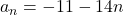Step-by-step explanation:

Given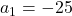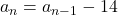Required

The explicit formula

First, calculate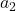Set n=2; So: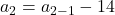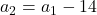Substitute -25 for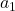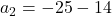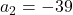Calculate the common difference (d)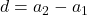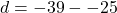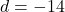So, the nth term is: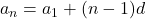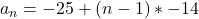Open bracket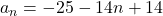Collect like terms